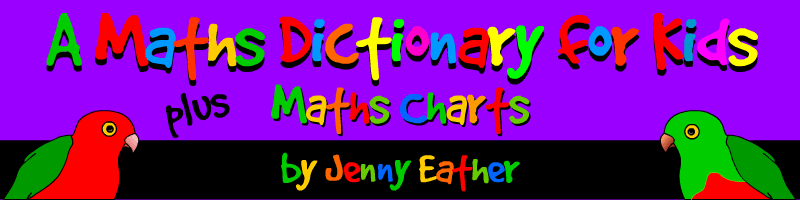::| FREE |::| PRINTABLE |:: A MATHS DICTIONARY FOR KIDS ~ DETAILS The original A Maths Dictionary for Kids is an animated, interactive online math dictionary for students which explains over 630 common mathematical terms and math words in simple language with definitions, examples, activities, practice and calculators. A Maths Dictionary for Kids Quick Reference is a device friendly html version with definitions and detailed examples for over 955 math words and terms.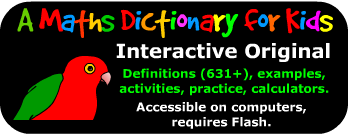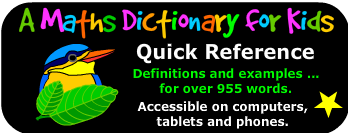> ENTER AMDFK DICTIONARY HERE < > ENTER AMDFK QR DICTIONARY HERE <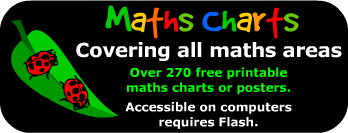> ENTER MATHS CHARTS HERE < > SEE MATHS CHARTS PDFs HERE < MATHS CHARTS ~ DETAILS Maths Charts is a collection of over 270 free printable maths posters suitable for interactive whiteboards, classroom displays, math walls, student handouts, homework help, introduction and consolidation of mathematical topics and other math reference needs. These free math posters include definitions and examples covering a wide range of math topics including Numbers, Operations on Numbers, Fractions, Decimals, Percent and Percentages, Ratios and Rates, Beginning Algebra, Data and Statistics, Probability, Geometry, Measurement, Time, Money, Symbols and Notation plus handy Blackline Masters. © Jenny Eather All rights reserved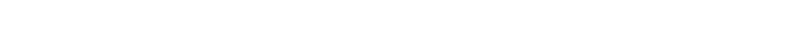::| Math Words | Math Terms | Math Glossary ::|:: Maths Words | Maths Terms | Maths Glossary |::
::| online math help | math dictionary for kids | free printable math posters | online maths help |::
::| printable math definitions ::|:: print posters and charts ::| :: print maths definitions |::
::| device friendly versions available |::